[Introduction]  [Signal arithmetic]  [Signals and noise]   [Smoothing]   [Differentiation]  [Peak Sharpening]  [Harmonic analysis]   [Fourier convolution]  [Fourier deconvolution]  [Fourier filter]  [Wavelets]   [Peak area measurement]  [Linear Least Squares]  [Multicomponent Spectroscopy]  [Iterative Curve Fitting]  [Hyperlinear quantitative absorption spectrophotometry] [Appendix and Case Studies]  [Peak Finding and Measurement]  [iPeak]   [iSignal]  [Peak Fitters]   [iFilter]  [iPower]  [List of downloadable software]  [Interactive tools]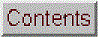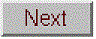Peak Sharpening (Resolution enhancement)

[Even derivative sharpening]  [First derivative symmetrization]  [The power law method]   [Combining sharpening methods]   [Spreadsheets]   [Matlab]   [Interactive tools]

The figure below shows a spectrum on the left that consists of several poorly-resolved (that is, partly overlapping) bands. The extensive overlap of the bands makes the accurate measurement of their intensities and positions impossible, even though the signal-to-noise ratio is very good. Things would be easier if the bands were more completely resolved, that is, if the bands were narrower.A peak sharpening algorithm has been applied to the signal on the left to artificially improve the apparent resolution of the peaks. In the resulting signal, right, the component bands are narrowed so that the intensities and positions can be measured, at the cost of a decrease in signal-to-noise ratio.

Here use can be made of peak sharpening (or resolution enhancement) algorithms to artificially improve the apparent resolution of the peaks. One of the simplest such algorithms is based on the weighted sum of the original signal and the negative of its second derivative:
Rj = Yj - k2Y''
where Rj is the resolution-enhanced signal, Y is the original signal, Y'' is the second derivative of Y, and k2 is a user-selected 2nd derivative weighting factor. It is left to the user to select the weighting factor k2 which gives the best trade-off between resolution enhancement, signal-to-noise degradation, and baseline flatness. The optimum choice depends upon the width, shape, and digitization interval of the signal. The result of the application of this algorithm is shown on the right. The component bands have been artificially narrowed so that the intensities and positions can be measured. The signal-to-noise ratio is degraded, but this can be moderated by smoothing, but at the expense of reducing the sharpening. Nevertheless, this technique will be useful only if the overlap of peaks rather than the signal-to-noise ratio is the limiting factor.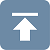Here's how it works. The figure below shows, in Window 1, a computer-generated peak (with a Lorentzian shape) in red, superimposed on the negative of its second derivative in green). (Click on the figure to see a full-size figure).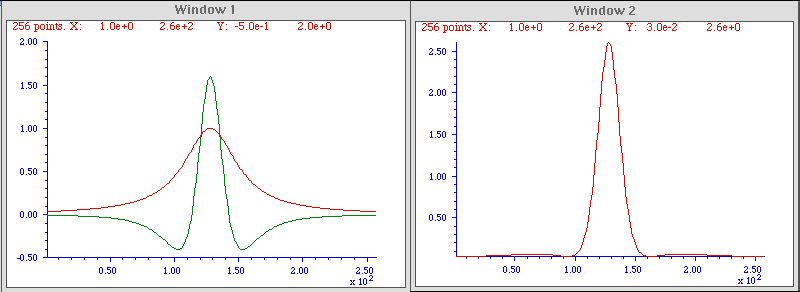Click to enlarge

The second derivative is amplified (by multiplying it by an adjustable constant) so that the negative sides of the inverted second derivative (from approximately X = 0 to 100 and from X = 150 to 250) are a mirror image of the sides of the original peak over those regions. In this way, when the original peak is added to the inverted second derivative, the two signals will approximately cancel out in the two side regions but will reinforce each other in the central region (from X = 100 to 150). The result, shown in Window 2, is a substantial (about 50%) reduction in the width, and a corresponding increase in height, of the peak. This effect is most dramatic with Lorentzian-shaped peaks; with Gaussian-shaped peaks, the resolution enhancement is less dramatic (only about 20 - 30%).The reduced widths of the sharpened peaks make it easier to distinguish overlapping peaks. In the example on the right, the raw signal (blue line) is actually composed of three overlapping Lorentzian peaks at x=200, 300, and 400, but the peaks are so wide - their halfwidths are 200, which is greater than their separation - that they blend together in the raw data, forming a wide asymmetrical peak with a maximum at x=220. The result of derivative sharpening (red line) clearly shows the underlying component peaks at their correct positions.

Note that the baseline of either side of the resolution-enhanced peak is not quite flat, especially for a Lorentzian peak, because the cancellation of the original peak and the inverted second derivative is only approximate; the adjustable weighting factor k is selected to minimize this effect. Peak sharpening will have little or no effect on the baseline, because if the baseline is linear, its derivative will be zero, and if it is gradually curved, its second derivative will be very small compared to that of the peak.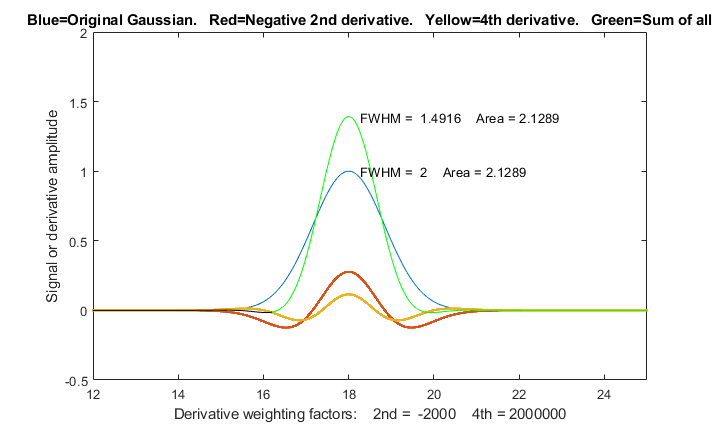This technique has been used in various forms of spectroscopy and chromatography for many years (references 74-76), even in some cases using analog electronics. Mathematically, the technique is a simplified version of a converging Taylor series expansion, in which only the even order derivative terms in the expansion are taken and for which their coefficients alternate in sign. The above example is the simplest possible version that includes only the first two terms - the original peak and its negative second derivative. Somewhat better results can be obtained by adding a fourth derivative term, with two adjustable factors k2 and k4:

Rj = Yj - k2Y'' + k4Y''''

where Y'' and Y'''' are the 2nd and 4th derivatives of Y. The result is a 21% reduction in width for a Gaussian peak, as shown in the figure on the left (Matlab/Octave script), and a 60% reduction for a Lorentzian peak (script). This is the algorithm that was used in the overlapping peak example above. (It's possible to add a sixth derivative term, but the series converges quickly and the results are only slightly improved, at the cost of increased complexity of three adjustable factors).

There is no universal optimum value for the derivative weighting factors; it depends on what you consider the best trade-off between peak sharpening and baseline flatness. However, a good place to start for a Gaussian peak are k2 = W2/32 and k4 = W4/900, and for Lorentzian peaks, k2=W2/4 and k4 = W4/600, where W is the halfwidth (FWHM) of the peak before sharpening, in x units. With those weighting factors, a Gaussian peak will be reduced in width by 21% and the resulting peak will still fit a Gaussian model with a percent fitting error of less than 0.3% and an R2 of 0.9999 (that is, very nearly a perfect fit). For a Lorentzian original shape, the peak width is reduced by a factor of 3, but the resulting peak fits a Gaussian model with a larger percent fitting error of 1.15% and an R2 of 0.9966.  Larger k values will result in a narrower peak, but the baseline on both side of the peak will exhibit a more pronounced negative undershoot. The software described below aids in the selection of the optimum degree of sharpening. Note that the K factors for the 2nd and 4th derivatives vary with the width raised to the 2nd and 4th power respectively, so they can vary over a very wide numerical range for peaks of different width. For this reason, if the peak widths vary substantially across the signal, it's possible to use segmented and gradient versions of this method so that the sharpening can be optimized for each region of the signal (see below).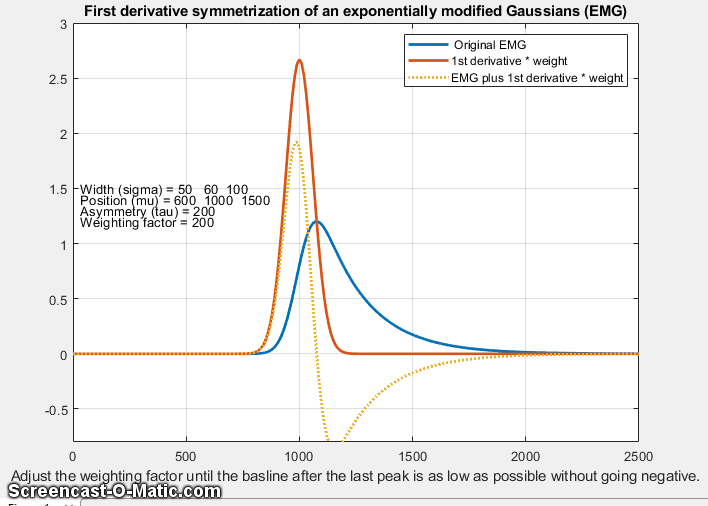First derivative symmetrization. The even-derivative technique described above works best for symmetrical peaks shapes. If the peak is asymmetrical - that is, slopes down faster on one side than the other - then the weighted addition (or subtraction) of a first derivative term, Y', may be helpful, because the first derivative of a peak is antisymmetric (positive on one side and negative on the other). This is also an old technique, having been used in chromatography since at least 1965 (reference 74), where it has been called "de-tailing". In fact, this works perfectly for exponentially broadened peaks of any shape (reference 70), for example the "exponentially modified Gaussian" (EMG) shape. In the graphic example on the right, the original peak (in blue) tails to the right, and its first derivative, Y', (dotted yellow) has a positive lobe on the left and a broader but smaller negative lobe on the right. When the EMG is added to the weighted first derivative, the positive lobe of the derivative reinforces the leading edge and the negative lobe suppresses the trailing edge, resulting in improved symmetry.
Sj = Yj + k1Y'j
With the correct first derivative weighting factor, k1, the result is a symmetrical Gaussian with a width substantially less than that of the original (orange line); in fact, it is exactly the underlying Gaussian to which the exponential convolution has been applied (References 70, 71). (Had the EMG sloped to the left, the negative of its derivative would be added). The first derivative weighting factor kis independent of the peak height and width and is simply equal to the exponential time constant tau (1/lambda) in some formulations of the EMG). It works perfectly for groups of peaks as long as the tau of each peak is the same. In practice k1 must be determined experimentally, which is most easily done for the last peak in a group of peaks (graphic, animation). Put simply, if you get k1 too high, the result will dip below the baseline after the peak. So it's easy to determine the optimal value experimentally; just increase it until the processed peak dips below the baseline after the peak, then reduce it until the baseline is flat, as shown in the GIF animation at this link. And if one stage of derivative addition does not do the trick, try one of the double exponential routines described below.

Furthermore, this appears to be a general behavior and it works similarly for any other peak shape that is broadened by exponential convolution, such a Lorentzian, and it even works for peaks that are already broadened by a previous exponential convolution (i.e a. double exponential). Such cases can be handled handled by two successive stages of derivative addition with different taus. (See
"double exponential symmetrization" below).

The symmetrized peak Sj resulting from thew first-derivative addition procedure can still be further sharpened by the even-derivative techniques described above, assuming that the signal-to-noise ratio of the original is good enough.

A useful property of all these derivative addition algorithms is that they do not change the total area under the peaks because the total area under the curve of any derivative of any peak-shaped signal is zero (the area under the negative lobes cancels the area under the positive lobes). Therefore these techniques can be helpful in measuring the areas under overlapped peaks. However, the problem is that the baseline on either side of the sharpened peak is not always perfectly flat, leaving some interference from nearby peaks, even if baseline resolution of adjacent peaks is achieved. For the even-derivative technique applied to a Lorentzian peak, about 80% of the area of the peak is contained in the central maximum, and for a Gaussian peak, over 99.7% of the area of the peak is contained in the central maximum.

Because differentiation and smoothing are both linear techniques, the superposition principle applies and the amplitude of a sharpened signal is directly proportional to the amplitude of the original signal, which allows quantitative analysis applications employing any of the standard calibration techniques). As long as you apply the same signal-processing techniques to the standards as well as to the samples, everything works.

Peak sharpening can be useful in automated peak detection and measurement to increase the ability to detect weak overlapping peaks that appear only as shoulders in the original signal. Click for animated example. Peak sharpening can be useful before measuring the areas under overlapping peaks, because it's easier and more accurate to measure the areas of peaks that are more completely separated.

The Power Law Method. An even simpler method for peak sharpening involves simply raising each data point to a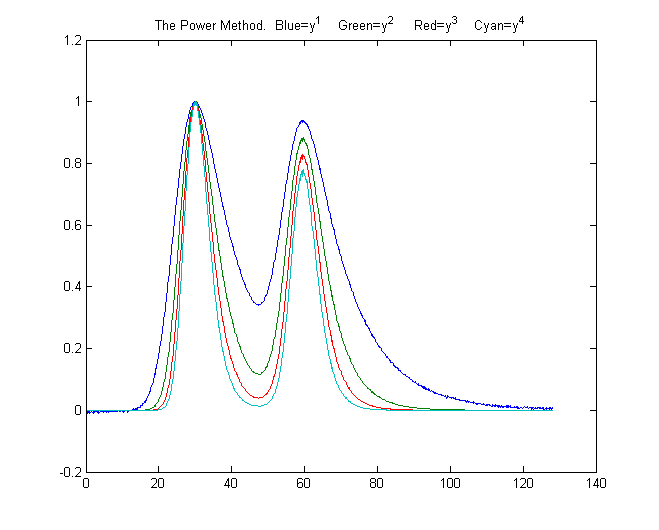power n greater than 1 (reference 61, 63). The effect of this is to change the peak shapes, essentially stretching out the highest center region of the peak to greater amplitudes and placi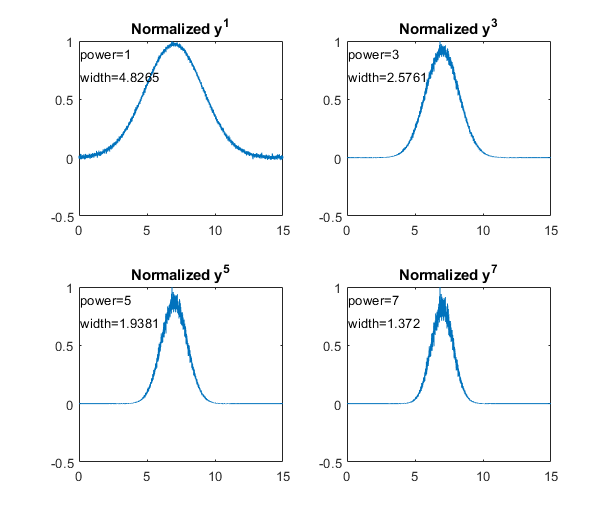ng more weight on the points near the peak, resulting in more nearly Gaussian peak shapes (because most peak shapes are locally Gaussian near the peak maximum) and smaller peak widths reducing the width by the square root of the power). The technique is demonstrated by the Matlab/Octave script PowerLawDemo.m, shown in the figure on the left, which plots noisy Gaussians raised to the power p=1 to 7, peak heights normalized to 1.0, showing that as the power increases, peak width decreases and noise is reduced on the baseline but increased on the peak maximum. Since the positions of the peaks are not moved by this process, the peak resolution (defined as the ratio of peak separation to peak width) is increased. In the figure on the right, the blue line shows two slightly overlapping peaks. The other lines are the result of raising the data to the power of n = 2, 3, and 4, and normalizing each to a height of 1.00. The peak widths, measured with the halfwidth.m function, are 19.2, 12.4, 9.9, and 8.4 units for powers 1 through 4, respectively. For Gaussian peaks, the area under the original peak can be calculated from the area under the normalized power-sharpened curve (reference 63). . However, there is a complication: for a signal of two overlapping Gaussians, the result of raising the signal to a power is not the same as adding two power-narrowed Gaussians: simply, an+bn is not the same as (a+b)n for n>1. This can be demonstrated graphically by the script PowerPeaks.m (graphic), which curve-fits a two-Gaussian model to the power-raised sum of two overlapping Gaussians; as the power n increases, the peaks are narrowed anda the valley between them deepened, but the resulting signal is no longer the sum of two Gaussians, unless the resolution is sufficiently high.

Some other limitations of the power law method:
1. it only works if the peaks of interest make a distinct maximum (it's not effective for side peaks that are so small that they only form shoulders; there must be a valley between the peaks);
2. the baseline must be zero for best results;
3. for noisy signals there is a decrease in signal-to-noise ratio because the smaller width means fewer data points are contributing to the measurement (smoothing can help).
4. the method introduces severe non-linearity into the signal, changing the ratios between peak heights (as is evident in the figure above right) and complicating further processing, especially quantitative measurement calibration.|

However, there is an easy way to compensate for this non-linearity in quantitative analysis application: after the raw data have been raised to the power n and peaks heights and/or areas have been measured, the resulting peak measures can be simply raised to the power 1/n, restoring the original linearity (but, notably, not the slope) of the calibration curves used in quantitative analytical measurements. (This works because the peak area is proportional to the height times width, and peak height of the power transformed peaks is proportional to the nth power of the original height, but the width of the peak is not a function of peak height at constant n, thus the area of the transformed peaks remains proportional to nth power of the original height). This technique is demonstrated quantitatively for two variable overlapping peaks by the Matlab/Octave script PowerLawCalibrationDemo.m (graphic) which takes the nth power of the overlapping-peak signal, measures the areas of the power-narrowed peaks, and then takes the 1/n power of the measured areas, constructing and using a calibration curve to convert areas to concentration. Peak areas are measured by perpendicular drop, using the half-way point to mark the boundary between the peaks. The script simulates a mixture signal with concentrations that you can specify in lines 15 and 16. You can change the power and any of the parameters in lines 14-22. The results show that the power method improves the accuracy of the measurements as long as the 4-sigma resolution (the ratio of peak separation to 4 times the sigma of the Gaussians) is above about 0.4. It is most accurate when the peaks are roughly equal in width and when the ratio of the two concentrations are not very different from the ratio in the standards from which the calibration curve is constructed. Note that, even when the random noise (in line 22) is zero, the results are not perfect due to effect of peak overlap on area measurement, which varies depending upon the ratio of two components in the mixture. (Requires gaussian.m, halfwidth.m, val2ind.m, and plotit.m downloaded from this web site).

The self-contained function demonstrates the power method for measuring the area of small shouldering peak that is partly overlapped by a much stronger interfering peak (Graphic). It shows the effect of random noise, smoothing, and any uncorrected background under the peaks.

Combining sharpening methods. The power method is independent of, and can be used in conjunction with, the derivative methods discussed above.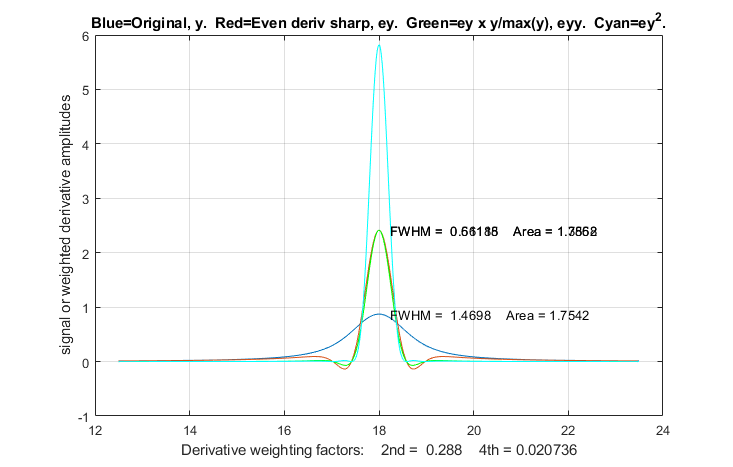However, because the power method is non-linear, the order in which the operations are performed is important. The first step should be the first-derivative symmetrization if the signal is exponentially broadened, the second step should be even-derivative sharpening, and the power method should be used last. The reason for this order is that the power method depends on, but can not create, a valley between highly overlapped peaks. The derivative methods may be able to create a valley between peaks if the overlap is not too severe. Moreover, when used last, the power method reduces the severity of baseline oscillations that are a residue of the even-derivative sharpening (particularly noticeable on a Lorentzian peak). The Matlab scripts SharpenedGaussianDemo2.m (Graphic) and SharpenedLorentzianDemo2.m (Graphic on right) make this point for Gaussian and Lorentzian peaks respectively, comparing the result of even-derivative sharpening alone with even-derivative sharpening followed by the power method (and preforming the power method two ways, taking the square of the sharpened peak or multiplying it by the original peak). For both the Gaussian and Lorentzian original peak shapes, the final sharpened results are fit to Gaussian models to show the changes in peak parameters. The result is that the combination of methods yields the narrowest final peak and the closest to Gaussian shape. Of course, the linearity issues of the power method, if used, remain.

Deconvolution. Another signal processing technique that can increase the resolution of overlapping peaks is deconvolution, which is treated in more detail here. It is applicable in the situation where the original shape of the peaks has been broadened and/or made asymmetrical by some broadening process or function. If the broadening process can be described mathematically or measured separately, then deconvolution from the observed broadened peaks is in principle capable of extracting the underlying peaks shape.

SPECTRUM, the freeware signal-processing application  for Mac OS8 and earlier, includes this resolution-enhancement algorithm, with adjustable weighting factor and derivative smoothing width.

Resolution Enhancement for Excel and Calc.
The even-derivative sharpening method with two derivative terms (2nd and 4th) is available for Excel and Calc (screen image) in the form of an empty template (PeakSharpeningDeriv.xlsx and PeakSharpeningDeriv.ods) or with example data entered (PeakSharpeningDerivWithData.xlsx and PeakSharpeningDerivWithData.ods). You can either type in the values of the derivative weighting factors K1 and K2 directly into cells J3 and J4, or you can enter the estimated peak width (FWHM in number of data points) in cell H4 and the spr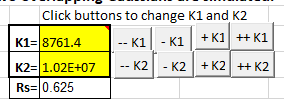eadsheet will calculate K1 and K2. There is also a demonstration version with adjustable simulated peaks which you can experiment with (PeakSharpeningDemo.xlsx and PeakSharpeningDemo.ods), as well as a version that has clickable ActiveX buttons (detail on left) for convenient interactive adjustment of the K1 and K2 factors by 1% or by 10% for each click.You can type in first estimates for K1 and K2 directly into cells J4 and J5 and then use the buttons to fine-tune the values. (Note: ActiveX buttons do not work in the iPad version of Excel). If the signal is noisy, adjust the smoothing using the 17 coefficients in row 5 columns K through AA, just as with the smoothing spreadsheets. There is also a 20-segment version where the sharpening constants can be specified for each of 20 signal segments (SegmentedPeakSharpeningDeriv.xlsx). For applications where the peak widths gradually increase (or decrease) with time, there is also a gradient peak sharpening template (GradientPeakSharpeningDeriv.xlsx) and an example with data (GradientPeakSharpeningDerivExample.xlsx); you need only set the starting and ending peak widths and the spreadsheet will apply the required sharpening factors K1 and K2.

The symmetrization of exponentially modified Gaussians (EMG) by the weighted addition of the first derivative is performed by the template PeakSymmetricalizationTemplate.xlsm (graphic). PeakSymmetricalizationExample.xlsm is an example application with sample data already typed in. There is also a demo version that simulates its own signal and allows you to determine the accuracy of the technique by internally generated overlapping peaks with specified resolution, asymmetry, relative peak height, noise and baseline: PeakSharpeningAreaMeasurementEMGDemo2.xlsm (graphic). These spreadsheets also allows further second derivative sharpening of the resulting symmetrical peak.

PeakDoubleSymmetrizationExample.xlsm performs the symmetrization of a doubly exponential broadened peak. It has buttons to interactively adjust the two first-derivative weighting. Two variations (1, 2) include data for two overlapping peaks, for which the areas are measured by perpendicular drop.

EffectOfNoiseAndBaselineNormalVsPower.xlsx demonstrates the effect of the power method on area measurements of Gaussian and exponentially broadened Gaussian peaks, including the different effect that random noise and non-zero baseline has on the power sharpening method. It shows that higher values of power (cell O9) reduce the peak width, makes the EMG peak more Gaussian, reduces the effect of the background (cell B6), and reduces the noise (cell B5) on the baseline but increases it near the peak maximum. Smoothing (cell B7) has little effect on the average peak area, but improves the reproducibility of the power method in the presence of noise.

Resolution Enhancement for Matlab and Octave
The custom Matlab/Octave function sharpen.m has the form SharpenedSignal = sharpen(signal,k1,k2,SmoothWidth), where "signal" is the original signal vector, the arguments k2 and k4 are 2nd and 4th derivative weighting factors, and SmoothWidth is the width of the built-in smooth. The resolution-enhanced signal is returned in the vector "SharpenedSignal". Click on this link to inspect the code, or right-click to download for use within Matlab or Octave. The values of k1 and k2 determine the trade-off between peak sharpness and baseline flatness; the values vary with the peak shape and width and should be adjusted for your own needs. For peaks of Gaussian shape, a reasonable value for k2 is PeakWidth2/25 and for k4 is PeakWidth4/800 (or PeakWidth2/6 and PeakWidth4/700 for Lorentzian peaks), where PeakWidth is the full-width at half maximum of the peaks. Because sharpening methods are typically sensitive to random noise in the signal, it's usually necessary to apply smoothing: the Matlab/Octave ProcessSignal.m function allows both sharpening and smoothing to be applied in one function.

Here's a simple example that creates a signal consisting of four partly-overlapping Gaussian peaks of equal height and width, applies both the derivative sharpening method and the power method, and compares a plot (shown below) comparing the original signal (in blue) to the resolution-enhanced version (in red):

x=0:.01:18;
y=exp(-(x-4).^2)+exp(-(x-9).^2)+exp(-(x-12).^2)+exp(-(x-13.7).^2);
y=y+.001.*randn(size(x));
k1=
1212;k2=1147420;
SharpenedSignal=ProcessSignal(x,y,0,35,3,0,1,k1,k2,0,0);
figure(1)
plot(x,y,x,SharpenedSignal,'r')
title('Peak sharpening (red) by the derivative method')
figure(2)
plot(x,y,x,y.^6,'r')
title('Peak sharpening (red) by the power method')

Four overlapping Gaussian peaks of equal height and width. Original: blue. After sharpening: red.
Left: Derivative method. Right: Power method

.SharpenedOverlapDemo.m is a script that automatically determines the optimum degree of even-derivative sharpening that minimizesthe errors of measuring peak areas of by the perpendicular drop method using the autopeaks.m function. It does this by applying different degrees of sharpening and plotting the area errors (percent difference between the true and measured errors) vs the sharpening factor, as shown on the right. It also shows the height of the valley between the peaks (yellow line). This demonstrates that (1) the optimum sharpening factor depends upon the width and separation of the two peaks and on their height ratio, (2) that the degree of sharpening is not overly critical, often exhibiting a broad optimum region, (3) that the optimum for the two peaks is not necessarily exactly the same, and (4) that the optimum for area measurement usually does not occur at the point where the valley is zero. (To run this script you must have gaussian.m, derivxy.m, autopeaks.m, val2ind.m, and halfwidth.m in the path. Download these from https://terpconnect.umd.edu/~toh/spectrum/).

The power method (right) is effective as long as there is a valley between the overlapping peaks, but it introduces non-linearity, which must be corrected later, whereas the derivative method preserves the original peak areas and the ratio between the peak heights. PowerLawCalibrationDemo demonstrates the linearization of the power transform calibration curves for two overlapping peaks by taking the nth power of data, locating the valley between them, measuring the areas by the perpendicular drop method, and then taking the 1/n power of the measured areas (graphic).

The symmetrize.m function. The symmetrization of asymmetric peaks by the weighted addition of the first derivative is performed by the function ySym = symmetrize(t,y,factor,smoothwidth,type,ends); "t" and "y" are the independent and dependent variable vectors, "factor" is the first derivative weighting factor,  and "smoothwidth", "type", and "ends" are the SegmentedSmooth parameters for the internal smoothing of the derivative. To perform a segmented symmetrization, "factor" and "smoothwidth" can be vectors. In version 2, symmetrize.m smooths only the derivative, not the entire signal. SymmetrizeDemo.m runs all five examples in the symmetrize.m help file, each in a different figure window.

First derivative symmetrization can be followed by an application of even derivative sharpening for further peak sharpening, as demonstrated for the exponentially modified Gaussians (EMG) by the self-contained Matlab/Octave function EMGplusfirstderivative.m and for the exponentially modified Lorentzian (EML) by EMLplusfirstderivative.m. In both of these, figure 1 shows the symmetrization and Figure 2 shows the additional 2nd and 4th derivative sharpening. Both of these routines report the before and after halfwidth and area of the peak, and they measure the resulting symmetry of the processed peak two ways: (a) by the ratio of the leading edge and trailing edge slopes (ideally -1.000) and (b) by the R2 of the least-squares fit to the initial Gaussian and Lorentzian shapes peak shape before exponential broadening respectively (ideally 1.000).SymmetizedOverlapDemo.m demonstrates the optimization of the first derivative symmetrization for the measurement of the areas of two overlapping exponentially broadened Gaussians, shown on the left. It plots and compares the original (blue) and sharpened peaks (red), then tries first-derivative weighting factors from +10% to -10% of the correct tau value in line 14) plots absolute peak area errors vs factor values. You can change the resolution by changing either the peak positions in  lines 17 and 18 or the peak width in line 13. Change height in line 16.  Must have derivxy.m, autopeaks.m, and halfwidth.m in the path.

Segmented derivative peak sharpening. If the peak widths vary substantially across the signal,you can use the segmented version SegmentedSharpen.m, for which the input arguments factor1, factor2, and SmoothWidth are vectors. The script DemoSegmentedSharpen.m, shown on the right, uses this function to sharpen four Gaussian peaks with gradually increasing peak widths from left to right with increasing degrees of sharpening, showing that the peak width is reduced by 20% to 22% compared to the original. DemoSegmentedSharpen2.m shows four peaks of the same width sharpened to increasing degrees. For asymmetrical peaks whose exponential broadening varies across the signal, you can use the symmetrize.m function described above in the same way: specify "factor" and "smoothwidth" as vectors, just like the segmented sharpening. And if peak widths and/or exponential factors increase or decrease regularly across the signal, you can simplify the calculation of these vectors by giving only the number of segments ("NumSegments"), the first value, "startv", and the last value, "endv", like so:

vstep=(endv-startv)/NumSegments;
vector=startv:vstep:endv;

where "vector" is the vector to use as an input argument for the symmetrize and SegmentedSharpen functions.

Double exponential symmetrization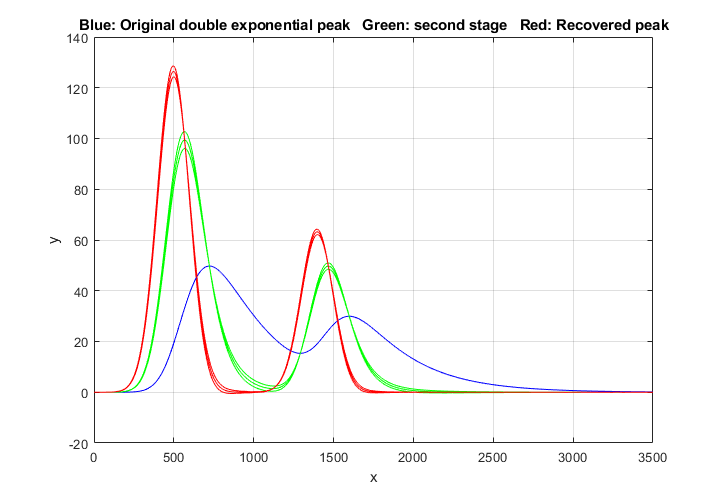in Matlab/Octave is performed by the function DEMSymm.m. It is demonstrated by the script DemoDEMSymm.m and its two variations (1, 2), which creates two overlapping double exponential peaks from Gaussian originals, then calls the function DEMSymm.m to perform the symmetrization, using a three-level plus-and-minus bracketing technique to help you to determine the best values of the two weighting factors by trial and error. In the example on the left, there are three red and three green bracketing lines produced by taus that are different by 10%; in this case the middle of the three bracketing lines is the optimum value.

If you attempt to symmetrize an asymmetrical peak with symmetrize.m, and the result is still asymmetrical, it's possible that the remaining asymmetry is at least approximately exponential with a different tau, so in that case the application of
DEMSymm.m will likely produce a more symmetrical final result.

ProcessSignal, a Matlab/Octave command-line function that performs smoothing, differentiation, and peak sharpening on the time-series data set x,y (column or row vectors). Type "help ProcessSignal". Returns the processed signal as a vector that has the same shape as x, regardless of the shape of y. The syntax is

Processed=ProcessSignal(x,y, DerivativeMode, w, type, ends,Sharpen, factor1, factor2, SlewRate, MedianWidth)

iSignal (Version 7) is a downloadable interactive multipurpose signal processing Matlab function that includes resolution enhancement for time-series signals, using both the even-derivative method (sharpen function)  and the first-derivative symmetrization method, with keystrokes that allow you to adjust the derivative weighting factors and the smoothing continuously while observing the effect on your signal dynamically. The E key turns the even-derivative resolution enhancement function on and off. View the code here or download the ZIP file with sample data for testing. iSignal calculates the sharpening and smoothing settings for Gaussian and for Lorentzian peak shapes using the Y and U keys, respectively, using the expression given above. Just isolate a single typical peak in the upper window using the pan and zoom keys, press P to your on the peakmeasurement mode, then press Y for Gaussian or U for Lorentzian peaks. You can fine-tune the sharpening with the F/V and G/B keys and the smoothing with the A/Z keys. (The optimum settings depends on the width of the peak, so if your signal has peaks of widely different widths, one setting will not be optimum for all the peaks. In such cases, you can use the segmented sharpen function,

Before Peak Sharpening in iSignal     After peak sharpening in iSignal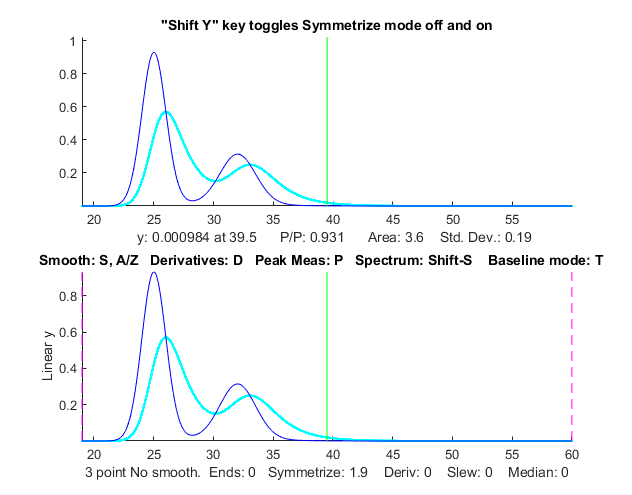If you have a signal that is exponentially broadened, you can remove that broadening (and increase the symmetry of the peaks) by the weighted first-derivative addition technique described here. Press Shift-Y and enter an estimated weighting factor (e.g., start with the width of the peak) then press "1" and "2" keys to change weighting factor by 10% and "Shift-1" and "Shift-2" keys to change by 1%. Increase the factor until the baseline after the peak goes negative, then increase it slightly so that it is a low as possible but not negative. Run the script iSignalSymmTest.m (graphic on left) for a example signal with two overlapping exponentially broadened Gaussians.

iSignal 5.95 can also use the power transform method (press the ^ key, enter the power, n (any positive number greater than 1.00) and press Enter.  To reverse this, simply raise to the 1/n power.

The latest version of iPeak, the Matlab interactive peak detection and measurement program,
now includes both the even-derivative method (sharpen function)  and the first-derivative symmetrization method, with keystrokes that allow you to adjust the derivative weighting factors and the smoothing continuously while observing the effect on your signal dynamically. The H key turns the even-derivative resolution enhancement function on and off. See ipeakdemo5. The de-tail (symmetrize) operation works as it does in iSignal, using the same Shift-Y, "1", "2", "Shift-1" and "Shift-2" keys.

Real-time peak sharpening in Matlab is discussed in Appendix Y.Last updated October, 2020. This page is part of "A Pragmatic Introduction to Signal Processing", created and maintained by Prof. Tom O'Haver , Department of Chemistry and Biochemistry, The University of Maryland at College Park. Comments, suggestions and questions should be directed to Prof. O'Haver at toh@umd.edu.
Unique visits since May 17, 2008: